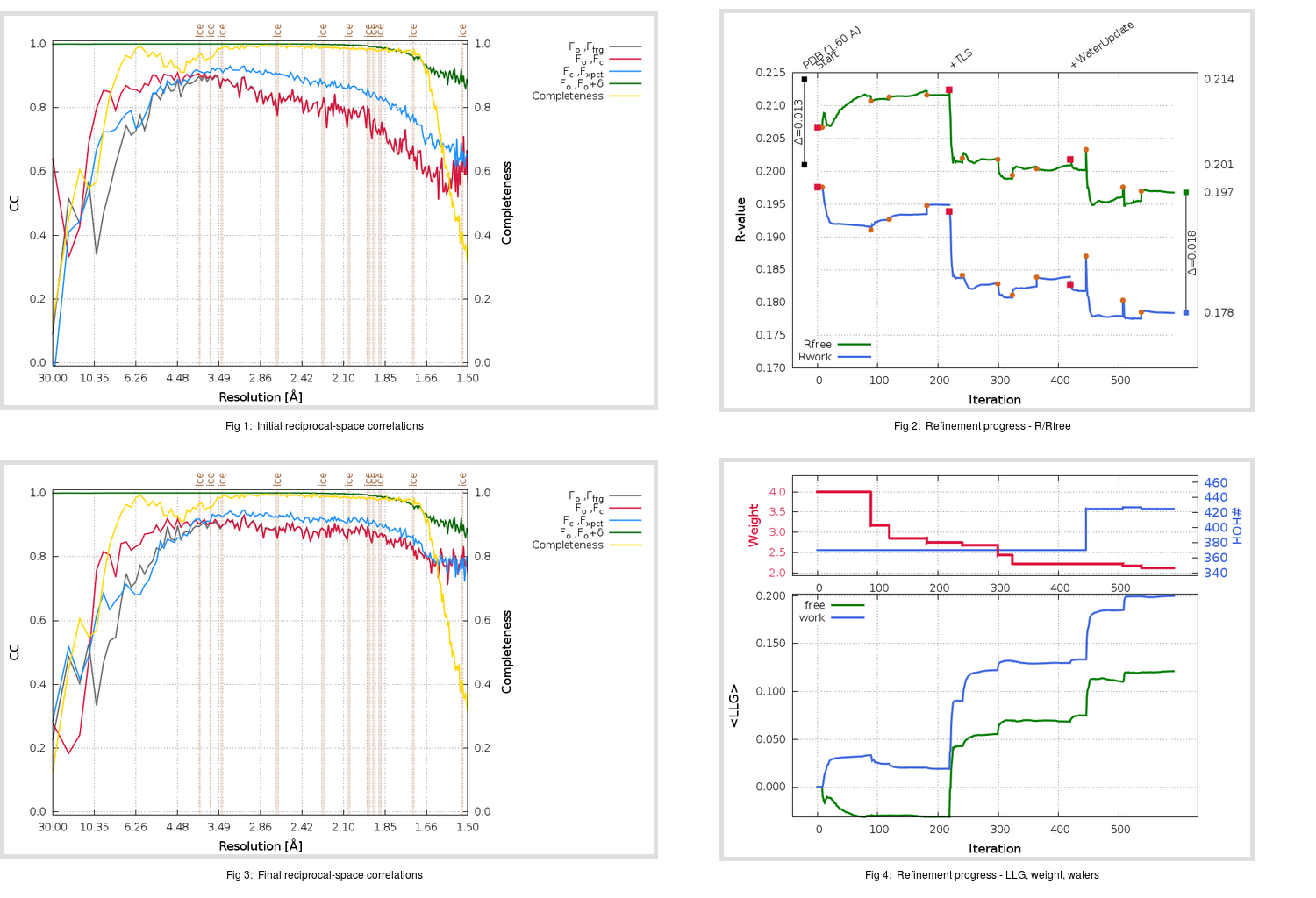Content:

```    Diffraction limits & principal axes of ellipsoid fitted to diffraction cut-off surface:
1.434         0.8860   0.0000  -0.4636       0.936 a* - 0.351 c*
1.567         0.0000   1.0000   0.0000       b*
1.586         0.4636   0.0000   0.8860       0.785 a* + 0.619 c*
```

## Deposited

` `
 Date deposited Date data collection Resolution R, Rfree 20060520 20060218 1.60 0.2010 0.2140

Molprobity (CCP4 7.0 version) summary:

```Ramachandran outliers =   0.00 %
favored =  99.01 %
Rotamer outliers      =   0.38 %
C-beta deviations     =     0
Clashscore            =   6.61
RMS(bonds)            =   0.0092
RMS(angles)           =   1.61
MolProbity score      =   1.36
Resolution            =   1.60
R-work                =   0.2010
R-free                =   0.2140
```

```Number of waters      =   370

<B> (all atoms) =   29.34 ( sd =    9.31 ) for       2741 non-hydrogen atoms
<B>   (protein) =   27.45 ( sd =    7.36 ) for       2371 non-hydrogen atoms
<B>     (water) =   41.50 ( sd =   11.31 ) for        370 non-hydrogen atoms
<B>    (others) =    0.00 ( sd =    0.00 ) for          0 non-hydrogen atoms

B min/max       (all non-hydrogen atoms) =   15.38 /   76.98
B min/max   (protein non-hydrogen atoms) =   15.38 /   62.80
B min/max     (water non-hydrogen atoms) =   18.06 /   76.98
B min/max     (other non-hydrogen atoms) =    0.00 /    0.00
```

## BUSTER (re-)refinement

` `

Molprobity (CCP4 7.0 version) summary:

```Ramachandran outliers =   0.00 %
favored =  98.68 %
Rotamer outliers      =   0.76 %
C-beta deviations     =     0
Clashscore            =   2.98
RMS(bonds)            =   0.0128
RMS(angles)           =   1.53
MolProbity score      =   1.09
Resolution            =   1.50
R-work                =   0.1784
R-free                =   0.1968
```

```Number of waters      =   425

<B> (all atoms) =   30.19 ( sd =   10.53 ) for       2796 non-hydrogen atoms
<B>   (protein) =   27.69 ( sd =    7.77 ) for       2371 non-hydrogen atoms
<B>     (water) =   44.20 ( sd =   12.86 ) for        425 non-hydrogen atoms
<B>    (others) =    0.00 ( sd =    0.00 ) for          0 non-hydrogen atoms

B min/max       (all non-hydrogen atoms) =   15.80 /   91.32
B min/max   (protein non-hydrogen atoms) =   15.80 /   69.96
B min/max     (water non-hydrogen atoms) =   17.91 /   91.32
B min/max     (other non-hydrogen atoms) =    0.00 /    0.00
```

Refinement progression:Results:

` `
 File Remark 2H2Z_aB_refine.01_03_refine.pdb.gz exact refinement commands are in header 2H2Z_aB_refine.01_03_refine.mtz.gz including original deposited data and several re-refinement map coefficients 2H2Z_aB_refine.01_03_BUSTER_model.cif.gz including any non-standard compound restraints 2H2Z_aB_refine.01_03_BUSTER_refln.cif.gz